Anzeige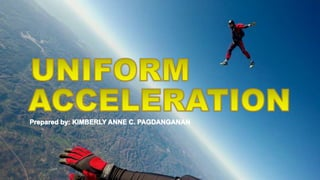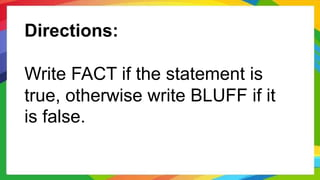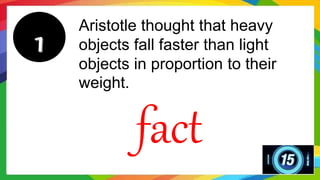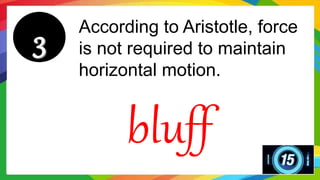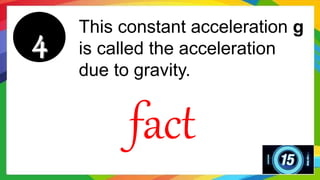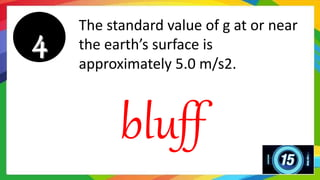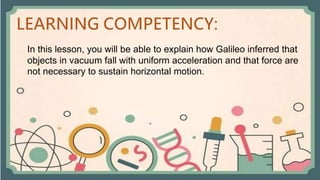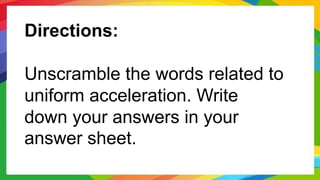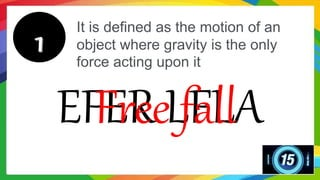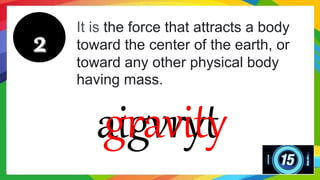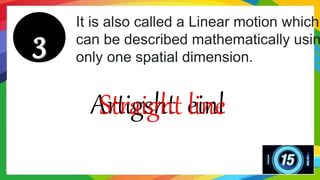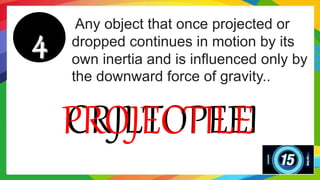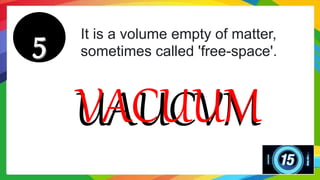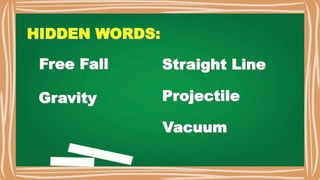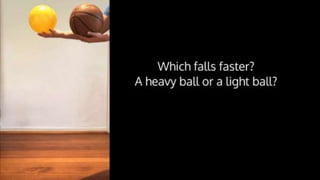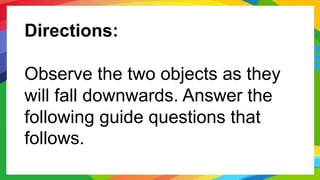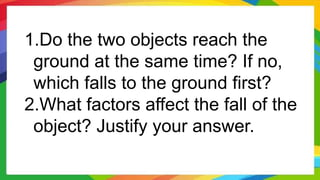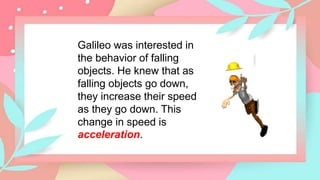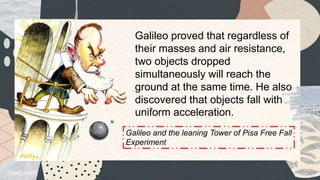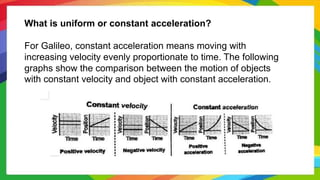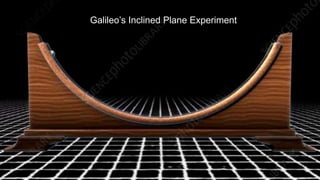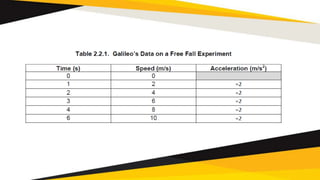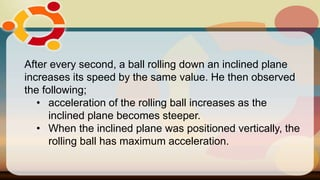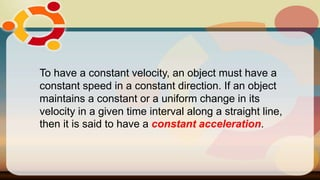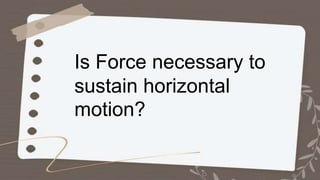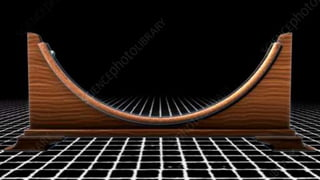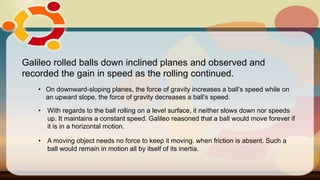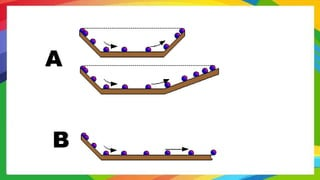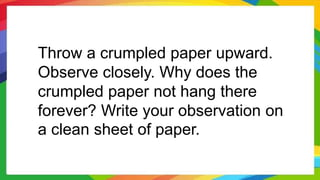1 von 35
Anzeige

### M2_Lesson 2_Uniform Acceleration.pptx

1. Directions: Write FACT if the statement is true, otherwise write BLUFF if it is false.
2. Aristotle thought that heavy objects fall faster than light objects in proportion to their weight. fact
3. Velocity is the length of the part travelled from starting point to final point bluff
4. According to Aristotle, force is not required to maintain horizontal motion. bluff
5. This constant acceleration g is called the acceleration due to gravity. fact
6. The standard value of g at or near the earth’s surface is approximately 5.0 m/s2. bluff
7. In this lesson, you will be able to explain how Galileo inferred that objects in vacuum fall with uniform acceleration and that force are not necessary to sustain horizontal motion.
9. It is defined as the motion of an object where gravity is the only force acting upon it EFER LFLA Free fall
10. It is the force that attracts a body toward the center of the earth, or toward any other physical body having mass. aigvryt gravity
11. It is also called a Linear motion which can be described mathematically usin only one spatial dimension. Artigsht einl Straight line
12. Any object that once projected or dropped continues in motion by its own inertia and is influenced only by the downward force of gravity.. CRJLTOPEEI PROJECTILE
13. It is a volume empty of matter, sometimes called 'free-space'. UAUCVM VACUUM
14. Directions: Observe the two objects as they will fall downwards. Answer the following guide questions that follows.
15. 1.Do the two objects reach the ground at the same time? If no, which falls to the ground first? 2.What factors affect the fall of the object? Justify your answer.
16. Galileo was interested in the behavior of falling objects. He knew that as falling objects go down, they increase their speed as they go down. This change in speed is acceleration.
17. Galileo proved that regardless of their masses and air resistance, two objects dropped simultaneously will reach the ground at the same time. He also discovered that objects fall with uniform acceleration. Galileo and the leaning Tower of Pisa Free Fall Experiment
18. What is uniform or constant acceleration? For Galileo, constant acceleration means moving with increasing velocity evenly proportionate to time. The following graphs show the comparison between the motion of objects with constant velocity and object with constant acceleration.
19. Galileo’s Inclined Plane Experiment
20. After every second, a ball rolling down an inclined plane increases its speed by the same value. He then observed the following; • acceleration of the rolling ball increases as the inclined plane becomes steeper. • When the inclined plane was positioned vertically, the rolling ball has maximum acceleration.
21. To have a constant velocity, an object must have a constant speed in a constant direction. If an object maintains a constant or a uniform change in its velocity in a given time interval along a straight line, then it is said to have a constant acceleration.
22. Is Force necessary to sustain horizontal motion?
23. Galileo rolled balls down inclined planes and observed and recorded the gain in speed as the rolling continued. • On downward-sloping planes, the force of gravity increases a ball’s speed while on an upward slope, the force of gravity decreases a ball’s speed. • With regards to the ball rolling on a level surface, it neither slows down nor speeds up. It maintains a constant speed. Galileo reasoned that a ball would move forever if it is in a horizontal motion. • A moving object needs no force to keep it moving. when friction is absent. Such a ball would remain in motion all by itself of its inertia.
24. A B
25. Throw a crumpled paper upward. Observe closely. Why does the crumpled paper not hang there forever? Write your observation on a clean sheet of paper.
Anzeige